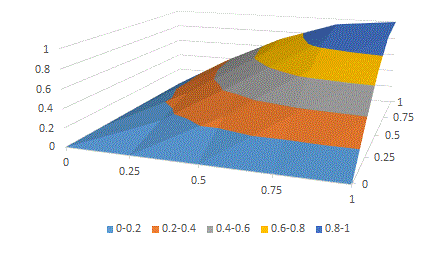Examples

 Example 1. Initial data, which were subject to approximation, were generated by the function sin(2x/π)sin(2y/π) with changing values of its argument on the interval [0 , 1] with the step 0.25 for x and y. Input file contained coordinates of 25 points: 0 0 0 0 0 0.25 0.25 0.25 0.25 0.25 0.5 0.5 0.5 0.5 0.5 0.75 0.75 0.75 0.75 0.75 1 1 1 1 1 0 0.25 0.5 0.75 1 0 0.25 0.5 0.75 1 0 0.25 0.5 0.75 1 0 0.25 0.5 0.75 1 0 0.25 0.5 0.75 1 0 0 0 0 0 0 0.146 0.271 0.354 0.383 0 0.27059805 0.5 0.653281482 0.707106781 0 0.353553391 0.653281482 0.853553391 0.923879533 0 0.382683432 0.707106781 0.923879533 1 Selected values qx=0, qy=0, ex=0, ey=0. Output:
Calculation #1. Thu Nov 10 12:34:08 EST 2022

Maximum error is 0.010421936533025946 at x=0.25 y=0.75
Standard deviation is 0.0017572929412511297

Approximation formula:
0.3185365XY/(1-0.948151X-0.95197Y+0.60672617X2+0.0026385237XY+0.608971Y2),
where X=x0.6 and Y=y0.6

Given and calculated data:

 x-coordinates y-coordinates Given z-coordinates Calculated z-coordinates 0.0 0.0 0.0 0.0 0.0 0.25 0.0 0.0 0.0 0.5 0.0 0.0 0.0 0.75 0.0 0.0 0.0 1.0 0.0 0.0 0.25 0.0 0.0 0.0 0.25 0.25 0.146 0.14958091 0.25 0.5 0.271 0.2692286 0.25 0.75 0.354 0.35021353 0.25 1.0 0.383 0.38493684 0.5 0.0 0.0 0.0 0.5 0.25 0.27059805 0.2689886 0.5 0.5 0.5 0.50140226 0.5 0.75 0.6532815 0.65494895 0.5 1.0 0.70710677 0.70724756 0.75 0.0 0.0 0.0 0.75 0.25 0.35355338 0.3498144 0.75 0.5 0.6532815 0.6547578 0.75 0.75 0.8535534 0.8554822 0.75 1.0 0.9238795 0.92152405 1.0 0.0 0.0 0.0 1.0 0.25 0.38268343 0.38459048 1.0 0.5 0.70710677 0.7071866 1.0 0.75 0.9238795 0.92169076 1.0 1.0 1.0 1.001386

Excel can be used for data visualizationBy selecting qx=1, qy=1, ex=1 ey=1 for the same input data, we had obtained the following

Calculation #2. Thu Nov 10 12:44:08 EST 2022

Maximum error is 0.03763636567674923 at x=0.25 y=0.25
Standard deviation is 0.00552270104131836247

Approximation formula:
(-0.0023584915+0.027621567X+0.02768925Y-0.027767392X2+1.4598988XY-0.027830912Y2)/(1-0.73942536X-0.7440697Y+0.71913296X2+0.4919999XY+0.7220544Y2),,
where X=x1.0 and Y=y1.0

 Example 2. Initial data, which were subject to approximation, were generated by the function (0.5 + Math.Sqrt(x) + y)/(1+2*Math.Sqrt(x)+0.5*y) Input file contained coordinates of 9 points: 1 1 1 2 2 2 3 3 3 1 2 3 1 2 3 1 2 3 0.714285714 0.875 1 0.673273103 0.810660172 0.922263446 0.65108474 0.774519053 0.877257154 Selected values qx=0, qy=0, ex=0, ey=0. This example (as many others) is used for validation of our software. Output:

Calculation #1. Thu Nov 10 14:27:37 EST 2022

Maximum error is 2.5738106484113824E-10 at x=2.0 y=1.0
Standard deviation is 1.1759820214120459E-10

Approximation formula:
(0.5+1.0X+1.0Y)/(1+2.0X+0.5Y),
where X=x0.5 and Y=y1.0

The obtained result coincides with the initial formula.